Kneser-Tits hypothesis

(diff) ← Older revision | Latest revision (diff) | Newer revision → (diff)
A conjecture on the structure of the-simple simply-connected algebraic groups that are isotropic over a field. Namely, the Kneser–Tits conjecture states that the group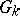of-rational points of a-simple simply-connected isotropic algebraic groupover a fieldis generated by its unipotent elements. This conjecture was stated in a somewhat less general form by M. Kneser; the general statement is due to J. Tits . For a group of type(see Semi-simple algebraic group) the Kneser–Tits conjecture is equivalent to the Tannaka–Artin problem: Does the group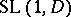of elements of reduced norm one of a finite-dimensional skew-fieldcoincide with the commutator subgroup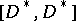of its multiplicative group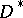? The Kneser–Tits conjecture has a close connection with questions of approximation in algebraic groups, rationality of group varieties and algebraic-theory.
The Kneser–Tits conjecture has been proved for the case of locally compact fields  and also for global function fields . Moreover, for global fields of characteristic zero the method of descent in  led to a proof of the Kneser–Tits conjecture for all algebraic groups except for those of types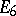and. However, the Kneser–Tits conjecture is not true in general, as follows from the negative solution to the Tannaka–Artin problem . As a result of this, progress has been made on the problem of the investigation of the measure of deviation offrom, which is expressible by the reduced Whitehead group (cf. also Linear group). The results obtained along these lines () form the basis of reduced-theory. It has been proved in  that the Kneser–Tits conjecture is also false for unitary groups, which in turn opens up a path for the development of reduced unitary-theory.